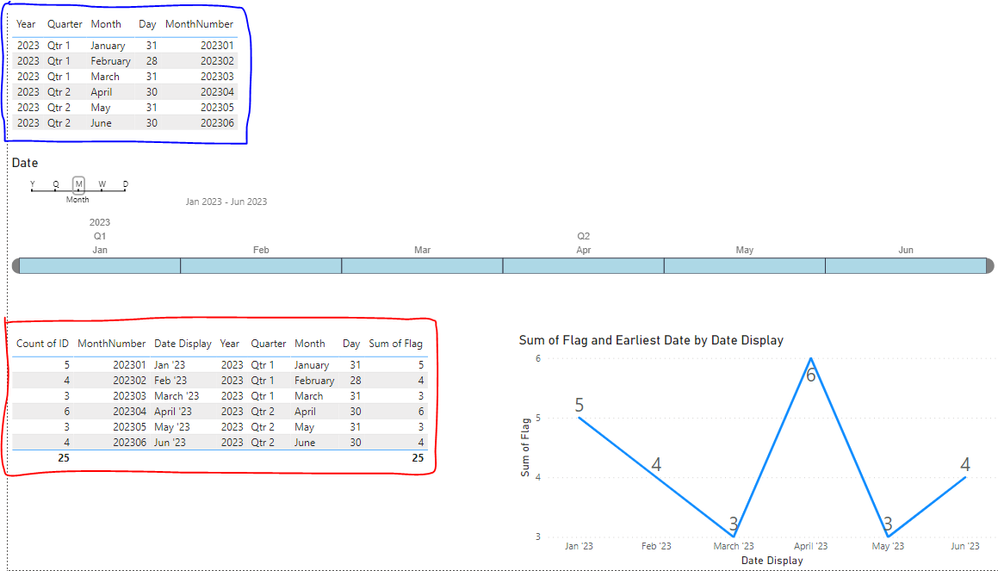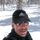cancel
Showing results for
Did you mean:

Fabric is Generally Available. Browse Fabric Presentations. Work towards your Fabric certification with the Cloud Skills Challenge.Helper IV

## Rolling average Last Month and Current Month

Hi,

I have two tables:

BLUE: Table used for Date slider/slicer
RED: Table used for all other dimensions/measuresI am plotting 'sum of flag' in a timeline.

I wish to plot rolling average of 'this month and last month' in the timeline.

That means for January: 5 is okay.
For February: it should be (5+4) / 2 = 4.5
For March: (4+3)/2 = 3.5

Thanks !

1 ACCEPTED SOLUTIONSuper User

Hi @Saniat ,

``````myMeasure =
VAR _monthNumberCurrent =    MAX ( mytable[monthNumber] ) //get the month number from this row

var _thisMonthFlag = MAX(myTable[Flag])
VAR _prevMonthNumber =
CALCULATE (
MAX ( mytable[monthNumber] ),
FILTER ( ALL ( myTable ), myTable[monthNumber] < _monthNumberCurrent )
) //filter the table to all month numbers less than this month number, and get the max value which is the month before

var _lastMonthFlag = CALCULATE(MAX(myTable[Flag]),FILTER(ALL(myTable),myTable[MonthNumber]=_prevMonthNumber))

RETURN
DIVIDE ( _thisMonthFlag + _lastMonthFlag, IF(_lastMonthFlag = 0,1,2 ))``````Let me know if you have any questions.

If this solves your issues, please mark it as the solution, so that others can find it easily. Kudos 👍are nice too.
Nathaniel

Proud to be a Super User!

4 REPLIES 4Super User

Hi @Saniat  @JohnShepherdAPD ,

``````myMeasure =
VAR _monthNumberCurrent =
MAX ( mytable[monthNumber] ) //get the month number from this row
VAR _prevMonthNumber =
CALCULATE (
MAX ( mytable[monthNumber] ),
FILTER ( ALL ( myTable ), myTable[monthNumber] < _monthNumberCurrent )
) //filter the table to all month numbers less than this month number, and get the max value which is the month before
RETURN
DIVIDE ( _monthNumberCurrent + _prevMonthNumber, 2 )
``````

Let me know if you have any questions.

If this solves your issues, please mark it as the solution, so that others can find it easily. Kudos 👍are nice too.
Nathaniel

Proud to be a Super User!Helper IV

Hi @Nathaniel_C ,

Thanks for this.

I am actually looking for average of [Flag] on those months ...

In the timeline on the right bottom corner ...
For February: it should be (5+4) / 2 = 4.5
For March: (4+3)/2 = 3.5

The final measure will be 2 months rolling average ...

Many thanksSuper User

Hi @Saniat ,

``````myMeasure =
VAR _monthNumberCurrent =    MAX ( mytable[monthNumber] ) //get the month number from this row

var _thisMonthFlag = MAX(myTable[Flag])
VAR _prevMonthNumber =
CALCULATE (
MAX ( mytable[monthNumber] ),
FILTER ( ALL ( myTable ), myTable[monthNumber] < _monthNumberCurrent )
) //filter the table to all month numbers less than this month number, and get the max value which is the month before

var _lastMonthFlag = CALCULATE(MAX(myTable[Flag]),FILTER(ALL(myTable),myTable[MonthNumber]=_prevMonthNumber))

RETURN
DIVIDE ( _thisMonthFlag + _lastMonthFlag, IF(_lastMonthFlag = 0,1,2 ))``````Let me know if you have any questions.

If this solves your issues, please mark it as the solution, so that others can find it easily. Kudos 👍are nice too.
Nathaniel

Proud to be a Super User!Helper II

Hi Saniat, take a look at the window function, some explanation is here:

https://www.sqlbi.com/articles/introducing-window-functions-in-dax/Announcements#### Power BI Monthly Update - November 2023

Check out the November 2023 Power BI update to learn about new features.#### Fabric Community News unified experience

Read the latest Fabric Community announcements, including updates on Power BI, Synapse, Data Factory and Data Activator.#### The largest Power BI and Fabric virtual conference

130+ sessions, 130+ speakers, Product managers, MVPs, and experts. All about Power BI and Fabric. Attend online or watch the recordings.Top Solution Authors
Top Kudoed Authors
Users online (2,615)